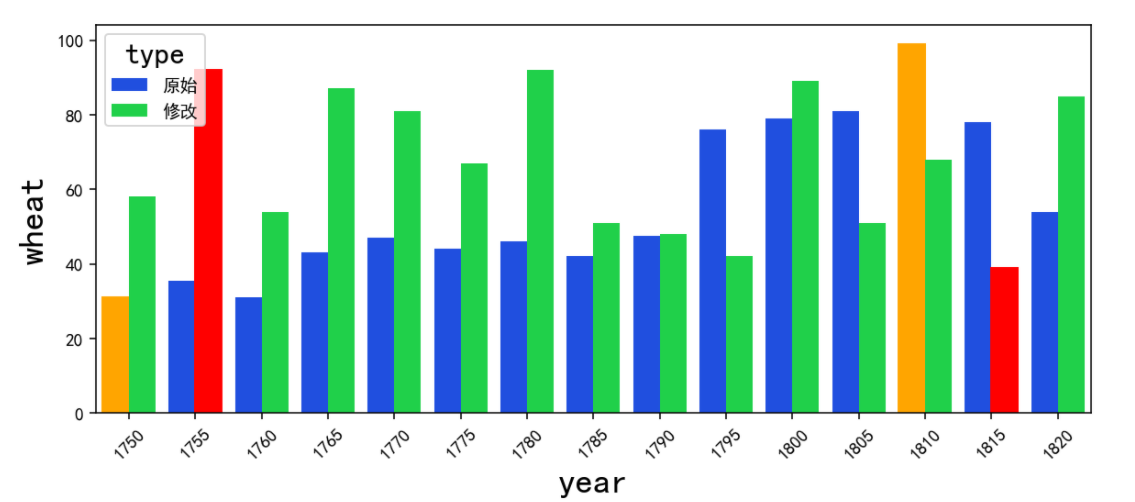• 写文章
• 提问

# python seaborn模块如何安装及使用？`pip install seaborn````import matplotlib.pyplot as plt
import seaborn as sns
%matplotlib inline
#sample方法
titanic.sample(10)```

# 通过'bins'参数设定数据片段的数量

`sns.distplot(age1,bins=30,kde=False)`

```import seaborn as sns
import matplotlib.pyplot as plt
%matplotlib inline